A Source Book in Mathematics, 1200-1800

Contents:
Author: Isaac Newton  | Date: 1945

Show Summary
Mathematics

5 NEWTON.

# Prime and Ultimate Ratios

### LEMMA I

Quantities, and the ratios of quantities, which in any finite time converge continually to equality, and before the end of that time approach nearer to each other than bt any given difference, become ultimately equal.

If you deny it, suppose them to be ultimately unequal, and let D be their ultimate difference. Therefore they cannot approach nearer to equality than by that given difference D; which is contrary to the supposition.

### LEMMA II

If in any figure AacE [Fig. 1], terminated by the right lines Aa, AE, and the curve acE, there be inscribed any number of parallelograms Ab, Bc, Cd, &c., comprehended under equal bases AB, BC, CD, &c., and the sides, Bb, Cc, Dd, &c., parallel to one side Aa of the figure; and the parallelograms aKbl, bLcm, cMdn,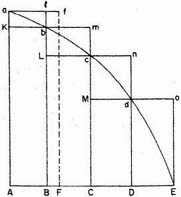Fig. 1

&c., are completed: then if the breadth of those parallelograms be supposed to be diminished, and their number to be augmented in infinitum, I say, that the ultimate ratios which the inscribed figure AKbLcMdD, the circumscribed figure AalbmcndoE, and curvilinear figure AabcdE, will have to one another, are ratios of equality.

For the difference of the inscribed and circumscribed figures is the sum of the parallelograms Kl, Lm, Mn, Do, that is (from the equality of all their bases), the rectangle under one of their bases Kb and the sum of their altitudes Aa, that is, the rectangle A Bla. But this rectangle, because its breadth AB is supposed diminished in infinitum, becomes less than any given space. And therefore (by Lem. 1) the figures inscribed and circumscribed become ultimately equal one to the other; and much more will the intermediate curvilinear figure be ultimately equal to either. Q.E.D.

### LEMMA III

The same ultimate ratios are also ratios of equality, when the breadths AB, BC, DC, &c., of the parallelograms are unequal, and are all diminished in infinitum.

For suppose AF equal to the greatest breadth, and complete the parallelogram F Aaf. This parallelogram will be greater than the difference of the inscribed and circumscribed figures; but, because its breadth AF is diminished in infinitum it will become less than any given rectangle. Q.E.D.

Corollary I. Hence the ultimate sum of those evanescent parallelograms will in all parts coincide with the curvilinear figure.

Corollary II. Much more will the rectilinear figure comprehended under the chords of the evanescent arcs ab, bc, cd, &c., ultimately coincide with the curvilinear figure.

Corollary III. And also the circumscribed rectilinear figure comprehended under the tangents of the same arcs.

Corollary IV. And therefore these ultimate figures (as to their perimeters acE) are not rectilinear, but curvilinear limits of rectilinear figures.

### LEMMA IV

If in two figures AacE, PprT [Fig. 2], there are inscribed (as before) two series of parallelograms, an equal number in each series, and, their breadths being diminished in infinitum, if the ultimate ratios of the parallelograms in one figure to those in the other, each to each respectively, are the same: I say, that those two figures, AacE, PprT, are to each other in that same ratio.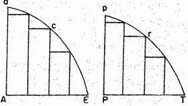Fig. 2

For as the parallelograms in the one are severally to the parallelograms in the other, so (by composition) is the sum of all in the one to the sum of all in the other; and so is the one figure to the other; because (by Lem. III) the former figure to the former sum, and the latter figure to the latter sum, are both in the ratio of equality. Q.E.D.

Corollary. Hence if two quantities of any kind are divided in any manner into an equal number of parts, and those parts, when their number is augmented, and their magnitude diminished in infinitum, have a given ratio to each other, the first to the first, the second to the second, and so on in order, all of them taken together will be to each other in that same given ratio. For if, in the figures of this Lemma, the parallelograms are taken to each other in the ratio of the parts, the sum of the parts will always be as the sum of the parallelograms; and therefore supposing the number of the parallelograms and parts to be augmented, and their magnitudes diminished in infinitum, those sums will be in the ultimate ratio of the parallelogram in the one figure to the correspondent parallelogram in the other; that is (by the supposition), in the ultimate ratio of any part of the one quantity to the correspondent part of the other.

### LEMMA V

All homologous sides of similar figures, whether curvilinear or rectilinear, are proportional; and the areas are as the squares of the homologous sides.

### LEMMA VI

If any are ACB [Fig. 3], given in position, is subtended by its chord AB, and in any point A, in the middle of the continued curvature, is touched by a right line AD, produced both ways; then if the points A and B approach one another and meet, I say, the angle BAD, contained between the chord and the tangent, will be diminished in infinitum, and ultimately will vanish.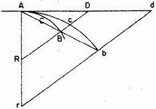Fig. 3

For if that angle does not vanish, the arc ACB will contain with the tangent AD an angle equal to a rectilinear angle; and therefore the curvature at the point A will not be continued, which is against the supposition.

### LEMMA VII

The same things being supposed, I say that the ultimate ratio of the arc, chord, and tangent, any one to any other, is the ratio of equality.1

For while the point B approaches towards the point A, consider always AB and AD as produced to the remote points b and d; and parallel to the secant BD draw bd; and let the arc Acb be always similar to the arc ACB. Then, supposing the points A and B to coincide, the angle dAb will vanish, by the preceding Lemma; and therefore the right lines Ab, Ad (which are always finite), and the intermediate arc Acb, will coincide, and become equal among themselves. Wherefore, the right lines AB, AD, and the intermediate arc ACB (which are always proportional to the former), will vanish, and ultimately acquire the ratio of equality. Q.E.D.

Corollary I. Whence if through B we draw BF parallel to the tangent, [Fig. 4], always cutting any right line AF passing through A in F, this line BF will be ultimately in the ratio of equality with the evanescent arc ACB; because, completing the parallelogram AFBD, it is always in a ratio of equality with AD.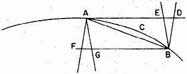Fig. 4

Corollary II. And if through B and A more right lines are drawn, as BE, BD, AF, AG, cutting the tangent AD and its parallel BF; the ultimate ratio of all the abscissas AD, AE, BF, BG, and of the chord and arc AB, any one to any other, will be the ratio of equality.

Corollary III. And therefore in all our reasoning about ultimate ratios, we may freely use any one of those lines for any other.

### LEMMA VIII

If the right lines AR, BR [Fig. 3], with the arc ACB, the chord AB, and the tangent AD, constitute three triangles RAB, RACB, RAD, and the points A and B approach and meet: I say, that the ultimate form of these evanescent triangles is that of similitude, and their ultimate ratio that of equality.

### LEMMA IX

If a right line AE [Fig. 5], and a curved line ABC, both given in position, cut each other in a given angle, A; and to that right line, in another given angle, BD, CE

are ordinately applied,2meeting the curve in B, C; and the points B and C together approach towards and meet in the point A: I say, that the areas of the triangles ABD, ACE, will ultimately be to each other as the squares of homologous sides.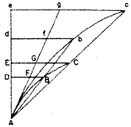Fig. 5

For while the points B, C, approach towards the point A, suppose always AD to be produced to the remote points d and e. so as Ad, Ae may be proportional to AD, AE; and the ordinates db, ec, to be drawn parallel to the ordinates DB and EC, and meeting AB and AC produced in b and c. Let the curve Abc be similar to the curve ABC, and draw the right line Ag so as to touch both curves in A, and cut the ordinates DB, EC, db, ec, in F, G, f, g. Then, supposing the length Ae to remain the same, let the points B and C meet in the point A; and the angle cAg vanishing, the curvilinear areas Abd, Ace will coincide with the rectilinear areas Afd, Age; and therefore (by Lemma V) will be one to the other in the duplicate ratio of the sides Ad, Ae. But the areas ABD, ACE are always proportional to these areas; and so the sides AD, AE are to these sides. And therefore the areas ABD, ACE are ultimately to each other as the squares of the sides AD, AE. Q.E.D.

### LEMMA X

The spaces which a body describes by any finite force urging it, whether that force is determined and immutable, or is continually augmented or continually diminished, are in the very beginning of the motion to each other as the squares of the times.

*

### SCHOLIUM

If in comparing with each other indeterminate quantities of different sorts, any one is said to be directly or inversely as any other, the meaning is, that the former is augmented or diminished in the same ratio as the latter, or as its reciprocal. And if any one is said to be as any other two or more, directly or inversely, the meaning is, that the first is augmented or diminished in the ratio compounded of the ratios in which the others, or the reciprocals of the others, are augmented or diminished. Thus, if A is said to be as B directly, and C directly, and D inversely, the meaning is, that A is augmented or diminished in the same ratio as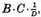that is to say, that A and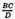are to each other in a given ratio.

### LEMMA XI

The evanescent subtense of the angle of contact, in all curves which at the point of contact have a finite curvature, is ultimately as the square of the subtense of the conterminous arc.3

Case 1. Let AB [Fig. 6] be that arc, AD its tangent, BD the subtense of the angle of contact perpendicular on the tangent, AB the subtense of the arc.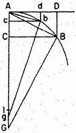Fig. 6

Draw BG perpendicular to the subtense AB, and AG perpendicular to the tangent AD, meeting in G; then let the points D, B, and G approach to the points d, b, and g, and suppose I to be the ultimate intersection of the lines BG, AG, when the points D, B have come to A. It is evident that the distance GI may be less than any assignable distance. But (from the nature of the circles passing through the points A, B, G, and through A, b, g),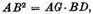and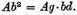But because GI may be assumed of less length than any assignable, the ratio of AG to Ag may be such as to differ from unity by less than any assignable difference; and therefore the ratio of AB2 to Ab2 may be such as to differ from the ratio of BD to bd by less than any assignable difference. Therefore, by Lem. I, ultimately,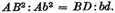Q.E.D.

Case 2. Now let BD be inclined to AD in any given angle, and the ultimate ratio of BD to bd will always be the same as before, and therefore the same with the ratio of AB2 to Ab2. Q.E.D.

Case 3. And if we suppose the angle D not to be given, but that the right line BD converges to a given point, or is determined by any other condition whatever; nevertheless the angles D, d, being determined by the same law, will always draw nearer to equality, and approach nearer to each other than by any assigned difference, and therefore, by Lemma I, will at last be equal; and therefore the lines BD, bd are in the same ratio to each other as before. Q.E.D.

Corollary I. Therefore since the tangents AD, Ad, the ares AB, Ab, and their sines, BC, bc, become ultimately equal to the chords AB, Ab, their squares will ultimately become as the subtenses BD, bd.

*Corollary II. Their squares are also ultimately as the versed sines of the arcs, bisecting the chords, and converging to a given point. For those versed sines are as the subtenses BD, bd.

*Corollary III. And therefore the versed sine is as the square of the time in which a body will describe the arc with a given velocity.

*Corollary IV. The ultimate proportion,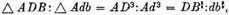is derived from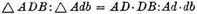and from the ultimate proportion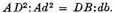So also is obtained ultimately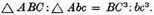Corollary V. And because DB, db are ultimately parallel and as the squares of the lines AD, Ad, the ultimate curvilinear areas ADB, Adb will be (by the nature of the parabola) two-thirds of the rectilinear triangles ADB, Adb, and the segments AB, Ab will be one-third of the same triangles. And thence those areas and those segments will be as the squares of the tangents AD, Ad, and also of the chords and arcs AB, AB.

### SCHOLIUM

But we have all along supposed the angle of contact to be neither infinitely greater nor infinitely less than the angles of contact made by circles and their tangents; that is, that the curvature at the point A is neither infinitely small nor infinitely great, and that the interval AJ is of a finite magnitude.

These Lemmas are premised to avoid the tediousness of deducing involved demonstrations ad sndutfum, according to the method of the ancient geometers. For demonstrations are shorter by the method of indivisibles; but because the hypothesis of indivisibles seems somewhat harsh, and therefore that method is reckoned less geometrical, I chose rather to reduce the demonstrations of the following Propositions to the first and last sums and ratios of nascent and evanescent quantities, that is, to the limits of those sums and ratios, and so to premise, as short as I could, the demonstrations of those limits. For hereby the same thing is performed as by the method of indivisibles; and now those principles being demonstrated, we may use them with greater safety. Therefore if hereafter I should happen to consider quantities as made up of particles, or should use little curved lines for right ones, I would not be understood to mean indivisibles, but evanescent divisible quantities; not the sums and ratios of determinate parts, but always the limits of sums and ratios; and that the force of such demonstrations always depends on the method laid down in the foregoing Lemmas.

Perhaps it may be objected, that there is no ultimate proportion of evanescent quantities; because the proportion, before the quantities have vanished, is not the ultimate, and when they are vanished, is none.5 But by the same argument it may be alleged that a body arriving at a certain place, and there stopping, has no ultimate velocity; because the velocity, before the body comes to the place, is not its ultimate velocity; when it has arrived, there is none. But the answer is easy; for by the ultimate velocity is meant that with which the body is moved, neither before it arrives at its last place and the motion ceases, nor after, but at the very instant it arrives; that is, that velocity with which the body arrives at its last place, and with which the motion ceases. And in like manner, by the ultimate ratio of evanescent quantities is to be understood the ratio of the quantities not before they vanish, nor afterwards, but with which they vanish. In like manner the first ratio of nascent quantities is that with which they begin to be. And the first or last sum is that with which they begin and cease to be (or to be augmented or diminished). There is a limit which the velocity at the end of the motion may attain, but not exceed. This is the ultimate velocity. And there is the like limit in all quantities and proportions that begin and cease to be. And since such limits are certain and definite, to determine the same is a problem strictly geometrical. But whatever is geometrical we may use in determining and demonstrating any other thing that is also geometrical.

It may also be objected, that if the ultimate ratios of evanescent quantities are given, their ultimate magnitudes will be also given: and so all quantities will consist of indivisibles, which is contrary to what Euclid has demonstrated conearning incommensurables, in the tenth Book of his Elements. But this objection is founded on a false supposition. For those ultimate ratios with which quantities vanish are not truly the ratios of ultimate quantities, but bruits towards which the ratios of quantities decreasing without limit do always converge; and to which they approach nearer than by any given difference, but never go beyond, nor in effect attain to, till the quantities are diminished in infinitum. This thing will appear more evident in quantities infinitely great. If two quantities, whose difference is given, be augmented in infinitum, the ultimate ratio of these quantities will be given, namely, the ratio of equality: but it does not from thence follow, that the ultimate or greatest quantities themselves, whose ratio that is, will be given. Therefore if in what follows, for the sake of being more easily understood, I should happen to mention quantities as least, or evanescent, or ultimate, you are not to suppose that quantities of any determinate magnitude are meant, but such as are conceived to be always diminished without end.

1 In our notation, lim arc ACB/chord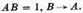2 Meaning "applied in order." From this expression, common at the time, our term ordinate is derived. See Selection V.1, note 1; also Selection IV.11, note 4.

3 A way of expressing this is that if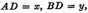the equation of the curve near A can be written in the form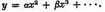4 Newton touches here upon the different types of angles of contact made by straight lines, tangent circles, and so on. Here he touches the age-old problem of the nature of hornlike angles (angtdi cornuti). It arose in connection with Proposition 16 of the third book of Euclid’s Elements: "The straight line drawn at right angles to the diameter of a circle from its extremity will fall outside the circle, and into the space between the straight line and the circumference another straight line cannot be interposed; further the angle of the semicircle is greater, and the remaining angle less, than every acute rectitineal angle." See T. L. Heath, The thirteen books of Euclid’s Elements (2nd ed.; Dover, New York, 1956), II, 39–43, where we find an account of the contributions to this question by Proclus, Cardan, Peletier, Clavins, Viète, and Wallis. See also F. Klein, Elementary mathematics from an advanced point of view, II (Macmillan, New York, 1939), 204, where it is shown that we are dealing here with non-Archimedeen quantities. See also E. Kasner, "The recent theory of the horn angle," Scripta Mathematica 11 (1945), 263–267.

5 Here we see Newton wrestling with the concept of "ultimate ratio of evanescent quantities" and Zeno’s reasoning. It was about this type of argument that Berkeley wrote his Analyst (Selection V.12). On Zeno’s argumentation and the debate around them during the centuries see F. Cajori, "The history of Zeno’s arguments on motion," American Mathematical Monthly 22 (1915), nine articles, and "The purpose of Zeno’s arguments on motion," Isis 3 (1920), 7–20. We now know—and it is even implicit in Newton—that the concept of limit cart be established without that of "ultimate ratio of evanescent quantities."

Contents:

### Related Resources

Sir Isaac Newton

Title: A Source Book in Mathematics, 1200-1800

Select an option:

## Email Options

Title: A Source Book in Mathematics, 1200-1800

Select an option: